Hello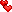| DE | Love the work of the JDs!
Forum posts by Rose342
 1. December 2023 15:25Everything else → Free gm to whoever guesses5081. December 2023 15:24Competitions and games → No vip? win 100 days!Wintery greetings8. October 2023 15:46Buy, sell and trade → Selling diamonds for sg'sI'd like to buy a few diamonds.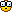What amount would you like to trade or have left?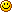Show postLast edited 8. October 2023 15:47 23. May 2023 14:03Buy, sell and trade → (S) 1st collection pantSobjection wrote:600 gmGive me a moment with WC.23. May 2023 13:56Buy, sell and trade → (S) 1st collection pantSobjection wrote:yeahfeel free to make a bid if IS is too high for yaalso selling 2 in blue and 9 in pink and grey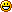highest bids1 black: 500 gm1 green: 400 gmIS: 9003 brown: 850 gm4 green: 500 gm4 pink: 1k+10 dia10 brown: 1500 gmIS: 2000 gm14: 700 gmIS: 1000 gmSOLD2 in green & grey812while you're here.. im also sellinghairshttps://gosupermodel.com/community/forum_thread.jsp?id=673359topshttps://gosupermodel.com/community/forum_thread.jsp?id=677829skirtshttps://gosupermodel.com/community/forum_thread.jsp?id=679214jacketshttps://gosupermodel.com/community/forum_thread.jsp?id=679386Nr 15?23. May 2023 13:55Competitions and games → guess the age21 Show postLast edited 23. May 2023 13:55 22. May 2023 16:02Everything else → should I risk it??Nope14. May 2023 21:24Competitions and games → let's level up together8. May 2023 12:20Everything else → i heard you can refund gsmWhimsibee wrote:i atleast feel scammed. Had i known i wouldnt have ever supported this place.I feel like I missed something crucial here - what happened?(My VIP is actually running out and thought about buying a new one.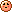) Show postLast edited 8. May 2023 12:21 19. April 2023 19:11Buy, sell and trade → free gocode itemsIf you want to get rid of the parrot, I'd love to get it.18. April 2023 19:17Buy, sell and trade → (S) 1st col, gopack, wanted +HouseOfDucks wrote:I'll send trade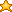Thank you18. April 2023 19:16Buy, sell and trade → (S) 1st col, gopack, wanted +HouseOfDucks wrote:250 for the sparkly one, 400 for the one with wingsIf that's an IS, you can send me a trade for both.Else you can put it down as an offer, if you want to wait for bids.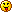18. April 2023 19:14Buy, sell and trade → (S) 1st col, gopack, wanted +What price was on your mind for those two dresses? https://gosupermodel.com/item/U4TTjrW-JxXR12wsqWLSNhttps://gosupermodel.com/item/xqyHuliy-jWa_dqRvyklP 18. April 2023 17:48Buy, sell and trade → (IS) old collection items <3ohroar wrote:yes!Thank you for the trade!18. April 2023 17:47Buy, sell and trade → (IS) old collection items <3Still selling 10?Show postLast edited 18. April 2023 17:47 15. April 2023 19:32Everything else → hey math nerd babesMimi wrote:The characteristic equation for the differential equation y'' - 3y = 0 is given by:r^2 - 3 = 0Solving for r, we get:r = +/- sqrt(3)Since the roots of the characteristic equation are real and distinct, the general solution for the differential equation is:y(x) = c1 e^(sqrt(3) x) + c2 e^(-sqrt(3) x)To find the solution in the surroundings of the point x=0, we can use a power series expansion of y(x) about x=0:y(x) = sum(n=0 to infinity) [a_n * x^n]Differentiating y(x) twice, we get:y''(x) = sum(n=2 to infinity) [n*(n-1) * a_n * x^(n-2)]Substituting these into the differential equation y'' - 3y = 0 and equating coefficients of like powers of x, we get:(n*(n-1) - 3) * a_n = 0Solving for a_n, we get:a_n = 0 for n=1 and n=2a_n = (3^(n/2) * c1 + (-3)^n/2 * c2) / (2^n * n!)Thus, the power series expansion of y(x) about x=0 is:y(x) = c1 + (sqrt(3)/2) * c1 x + (1/4) * (3/2) * c1 x^2 + (sqrt(3)/8) * (3/2) * c1 x^3 + ...+ c2 + (-sqrt(3)/2) * c2 x + (1/4) * (-3/2) * c2 x^2 + (-sqrt(3)/8) * (-3/2) * c2 x^3 + ...Since the differential equation is linear, the power series solution y(x) is also the unique real analytic solution in the surroundings of the point x=0.So this would be your way to go. I didn't check the solution, though, since it would actually mean getting a paper and solving the exercise.So basically: 1. You're writing y(x) = _ as a power series, not sure if you had the same approach as we did there.2. You're differentiating this twice, so you get a solution for y''(x) = _3. In the equation y''(x) - 3 y(x) = 0, you're changing y(x) = _ and y''(x) = _ to the solutions calculated in 1. and 2. Sort the terms afterwards. 4. Coefficient comparison Show postLast edited 15. April 2023 19:40 15. April 2023 19:29Everything else → hey math nerd babesshybi wrote:yeah i don't have any other info. but isn't this just a general solution everywhere, not just around x = 0? i'm not anymore sure what is real analytic/power series solution and should i do it with sums like i did in other problem.Can you maybe type the exact wording of the exercise?It's kinda hard with english since I don't know all the terms too.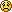Edit: Wait, I just inserted your english term in a translator. Power series would mean to solve it with the approach with the rows.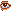Show postLast edited 15. April 2023 19:30 15. April 2023 19:03Everything else → hey math nerd babesshybi wrote:yes plsi have a lot of these problems. but for the problem i put here, sure. i'm trying to understand the chatgpt one if it's correctThe ChatGPT one is correct, although I think it might be hard to understand if you don't already now what you're trying to get. Your problem is a second-order linear homogenous differential equation, this is correct.There are different options to solve it, the one suggested from ChatGPT is for the given example probably the best one and we called it "constant coefficients". (Not sure if it's the same in your language. However, someone mentioned a solution with rows that should also work. I wouldn't use it for the given problem, however, far too complex.) If you know the quation is homogenous and linear, you can transform the given y''(x) - 3*y(x) = 0 into the characteristic equation r^2 - 3 = 0.From this, you're trying to get the zeropoints. Since it is a binomial equation, you can see them basically: +/- sqrt(3). So the zeropoints are reel and not complex and both are with a multiplicity of 1.With this knowledge, you can create the general solution, that also was given with ChatGPT: y(x) = C1 * e^(sqrt(3) * x) + C2 * e^(-sqrt(3) * x)I'm not sure if you've been given an additional information to solve the problem with a particular solution. Did you get any more information like y(0) = _?That's what the last paragraph of ChatGPT is about. If you've been given more information, you use this to calculate the constants c1 and c2. Show postLast edited 15. April 2023 19:09 15. April 2023 18:34Everything else → hey math nerd babesshybi wrote:can you help me with my math problem?i have to find a real analytic (power series may be the correct term??) solution in the surroundings of the point x = 0 for a function y''-3y=0.and no, i'm not sure if this is correct in english but i tried.Is help still needed?15. April 2023 18:25Everything else → People who complainedmeriharakka wrote:wtf did someone actually whine about it being too easyYes, yesterday loads of people complained about it being too easy. Guess the "update" is now the new difficulty.